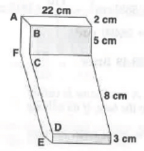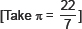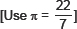# Surface Areas and Volumes-Sample Questions

Class X Math
Sample Paper for Surface Areas and Volumes
1.   A toy is in the form of a cone mounted on a hemisphere with same radius. The diameter of the base of the conical portion is 7 cm and the total height of the toy is 14.5 cm. Find the volume of the toy.
2.   In the figure, the shape of a solid copper piece (made of two pieces) with dimensions as shown. The face ABCDEFA has uniform cross section. Assume that the angles at A, B, C, D, E and F are right angles. Calculate the volume of the piece.3.   A toy is in the form of a cone mounted on a hemisphere of common base radius 7 cm. The total height of the toy is 31 cm. Find the total surface area of the toy.4.   A toy is in the form of a cone mounted on a hemisphere of common base of diameter 7 cm. If the height of the toy is 15.5 cm, find the total surface area of the toy.5.   A circular tent is cylinderical up to a height of 3 m and conical above it. If the diameter of the base of the cone and cylinder is 105 m and the slant height of the conical part is 53 m, find the total canvas used in making the tent.
6.   A solid composed of a cylinder with hemispherical ends. if the whole length of the solid is 108 cm and the diameter of the hemispherical end is 36 cm, find the cost of polishing its surface at the rate of 70 paise per square cm.
7.   A 5 m wide cloth is used to make a conical tent of base diameter 14m and height 24m. Find the cost of cloth used at the rate of Rs. 25 per metre.8.   A girl empties a cylinderical bucket, full of sand, of base radius 18 cm and height 32 cm, on the floor to form a conical heap of sand. If the height of this conical heap is 24 cm, then find its slant height correct up to one place of decimal.
9.   The largest possible sphere is carved out of a wooden solid cube of side 7 cm. Find the volume of the wood left.10.   150 spherical marbles, each of radius 1.4 cm, are dropped in a cylinderical vessel fo radius 7 cm containing some water, which are completely immersed in water. Find the rise in the level of water in the vassel.